# ##智能优化算法复习--免疫算法IA

1年前   阅读数 168 0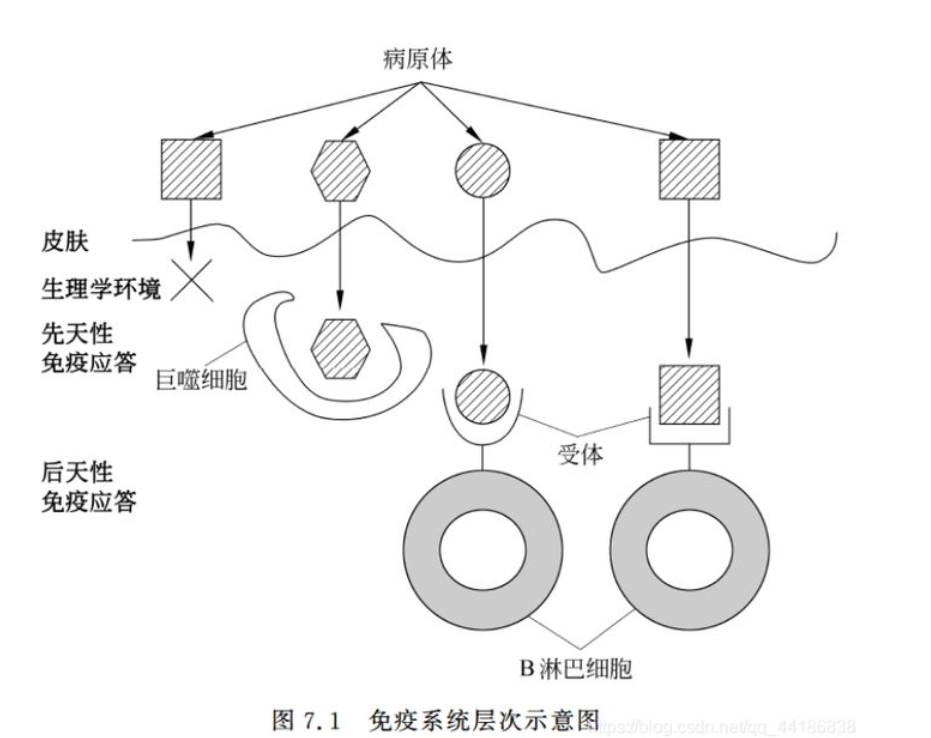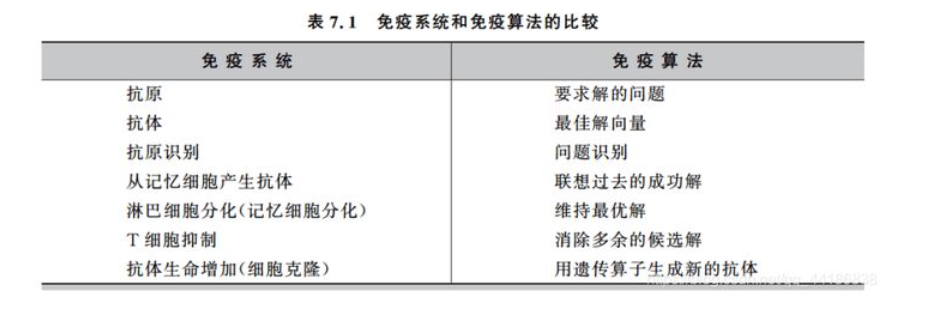(1)抗原识别与初始抗体的产生

（2）抗体评价

（3）免疫操作（利用免疫选择，克隆，变异，克隆抑制，种群刷新等算子模拟生物免疫）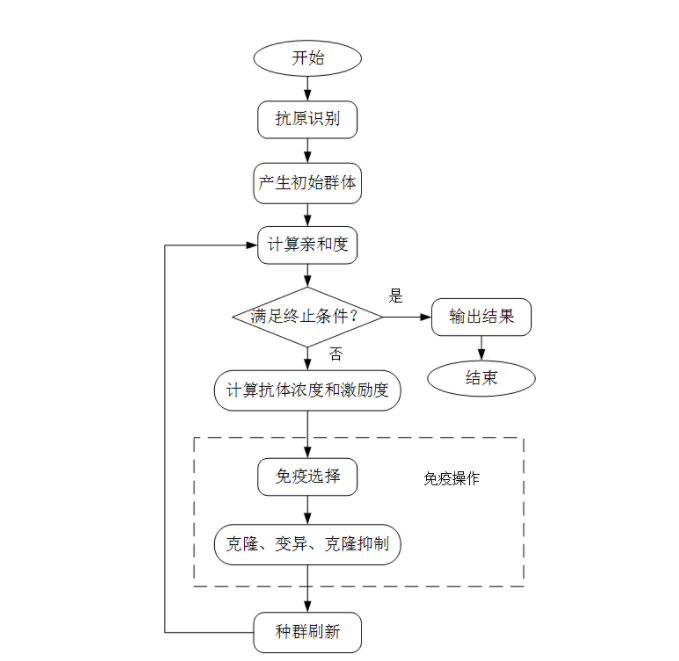1、基本步骤：

（1）首先进行抗原识别，即理解代优化问题，构造合适的亲和度函数及各种约束条件。

（2）生成初始种群

（3）对种群中的每一个个体进行亲和度评价

（4）判断算法是否满足终止条件，如果满足则算法终止，输出计算结果；否则，继续寻优计算

（5）计算抗体浓度和激励度

（6）进行免疫处理，包括免疫选择、克隆、变异和克隆抑制

（7）种群刷新，以随机生成的新抗体替代种群中激励度较低的抗体，形成新一代抗体，转

2.  关键参数

抗体种群大小NP：10~100，一般不超过200

免疫选择比例：一般取抗体种群大小的10%~50%

抗体克隆扩增的倍数：一般取5~10倍

种群刷新比例：每代更新的抗体一般不超过抗体种群的50%

最大进化代数G:一般100-500

3、算子

（1）亲和度评价算子

通常函数优化问题可以用函数值或对函数值的简单处理（如取倒数、相反数等）作为亲和度评价，而对于组合优化问题或其它问题，则需具体问题具体分析，通常是一个函数：aff(x)。在这可以取1/（1+目标函数）来作为亲和度函数，更多的还是具体问题具体分析。

（2）抗体浓度评价算子

抗体浓度通常定义为：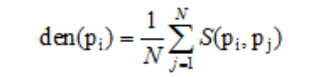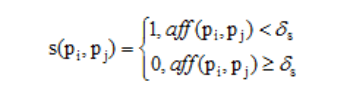抗体间亲和度的计算方法主要包括基于抗体和抗原亲和度的计算方法、基于欧式距离的计算方法、基于海明距离的计算方法、基于信息熵的计算方法等。

1、基于欧式距离的抗体间亲和第计算方法

对于实数编码的算法，抗体间亲和第通常可以通过抗体向量之间的欧式距离来计算：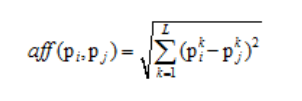其中，pik为抗体i的第k维度，L是抗体编码的总维数。

***举个例子说明一下：比如有两个四维的抗体，那么他们的抗体间亲和度怎么算呢，

2、基于海明距离的抗体间亲和度计算方法

对于基于离散编码的算法，衡量抗体-抗体亲和度最直接的方法就是利用抗体串的海明距离：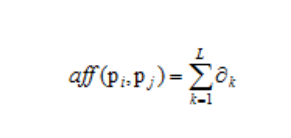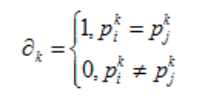***举个例子说明一下，比如有两个四维的抗体，

（3）激励度计算算子

抗体激励度是对抗体质量的最终评价结果，通常亲和度大、浓度低的抗体会得到较大的激励度。抗体激励度的计算通常如下所示：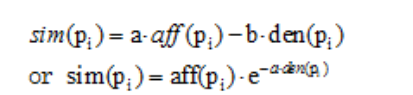（4）免疫选择算子

根据抗体的激励度确定哪些抗体被选择进入克隆选择操作。一般，激励度高的抗体更可能被选中

（5）克隆算子

克隆算子将免疫选择算子选中的抗体进行复制。其可描述为：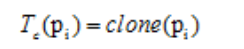（6）变异算子

1、实数编码算法变异算子

实数变异算子的变异策略是在变异源个体中加入一个小扰动。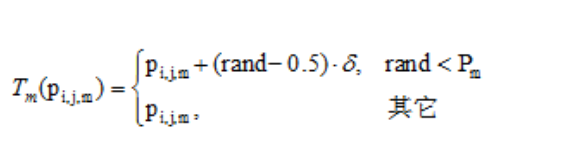2、离散编码算法变异算子

离散编码算法以二进制编码为主，其变异策略是从变异源抗体串中随机选取几位元，改变位元的取值（取反）。

（7）克隆抑制算子

克隆抑制算子用于对经过变异后的克隆体进行再选择，抑制亲和度低的抗体，保留亲和度高的抗体进入新的抗体种群。

（8）种群刷新算子

对种群中激励度较低的抗体进行刷新，从抗体种群中删除这些抗体并以随机生成的新抗体替代。

下面来展示一个具体实例

``````%%%%%%%%%%%%%%%%%%%%免疫算法求解TSP%%%%%%%%%%
%%%%%%%%%%%%初始化%%%%%%%%%%%%%%%%%%%%%%%%%
clear all;
close all;
clc;
c = [1304 2313;3639 1315;4177 2244;3712 1399;3488 1535;3326 1556;
2788 1229;4196 1044;4312 790 ;4386 570 ;3007 1970;2562 1756;
2788 1491;2381 1676;1332 695; 3715 1678;3918 2179;4061 2370;
3780 2212;3676 2578;4029 2838;4263 2931;3429 1908;3507 2376;
3394 2643;3439 3201;2935 3240;3140 3550;2545 2357;2778 2826;
2370 2975];
N = size(c,1);
D = zeros(N);
%%%%%%%%%%%%%%%%%%%%%%%%%%%求任意两个城市距离间隔矩阵%%%%%%
for i=1:N
for j=1:N
D(i,j)=((c(i,1)-c(j,1))^2+(c(i,2)-c(j,2))^2)^0.5;
end
end
NP=200;            %免疫个体数目
G=1000;        %最大免疫代数
f=zeros(N,NP);  %用于存储种群
for i=1:NP
f(:,i)=randperm(N);  %随机生成初始种群
end
len=zeros(NP,1);      %存储路径长度
for i=1:NP
len(i)=func3(D,f(:,i),N);  % 计算路径长度
end
[Sortlen,Index]=sort(len);
Sortf=f(:,Index);            %种群按个体排序
gen=0;                       %免疫代数
Ncl=10;                      %克隆次数
%%%%%%%%%%%%%%%%%%%%%免疫循环%%%%%%%%%%%%
while gen<G
for i=1:NP/2
%%%%%选激励度前NP/2个个体进行免疫操作%%%%%%%%%
a=Sortf(:,i);
Ca=repmat(a,1,Ncl);
for j=1:Ncl
p1=floor(1+N*rand());
p2=floor(1+N*rand());
while p1==p2
p1=floor(1+N*rand());
p2=floor(1+N*rand());
end
tmp=Ca(p1,j);
Ca(p1,j)=Ca(p2,j);
Ca(p2,j)=tmp;
end
Ca(:,1)=Sortf(:,i);
%%%%%%%%%%克隆抑制，保留亲和度最高的个体%%%%%
for j=1:Ncl
Calen(j)=func3(D,Ca(:,j),N);
end
[SortCalen,Index]=sort(Calen);
SortCa=Ca(:,Index);
af(:,i)=SortCa(:,1);
alen(i)=SortCalen(1);
end
%%%%%%%%%%%%%%%%种群刷新%%%%%%%%%%%%%%%
for i = 1:NP/2
bf(:,i) = randperm(N);
blen(i) = func3(D,bf(:,i),N);
end
%%%%%%%%%%%%%%%%%%免疫种群与新种群合并%%%%%%%%%%
f = [af,bf];
len = [alen,blen];
[Sortlen,Index] = sort(len);
Sortf = f(:,Index);
gen = gen + 1;
trace(gen) = Sortlen(1);
end

%%%%%%%%%%%%%%输出优化结果%%%%%%%%%%%%
Bestf = Sortf(:,1);
Bestlrn = trace(end);
figure
for i = 1:N - 1
plot([c(Bestf(i),1),c(Bestf(i + 1),1)],...
[c(Bestf(i),2),c(Bestf(i + 1),2)],'bo-');
hold on ;
end
plot([c(Bestf(N),1),c(Bestf(1),1)],...
[c(Bestf(N),2),c(Bestf(1),2)],'ro-');
title(['优化最短距离：',num2str(trace(end))]);
figure,plot(trace)
xlabel('迭代次数')
ylabel('目标函数值')
title('亲和度进化曲线')
%%%%%%%%%%计算路线总长度%%%%%%%%%%%%%%%%%
function len = func3(D,f,N)
len = D(f(N),f(1));
for i = 1:(N - 1)
len = len + D(f(i),f(i + 1));
end
end
``````

我有话说: